• Home   |
• Privacy   |
• Shop   |
• 🔍 Search Site
• Halloween Color By Number
• Halloween Dot to Dot
• Kindergarten Halloween Sheets
• Puzzles & Challenges for Older Kids
• Kindergarten Thanksgiving
• Christmas Worksheets
• Easter Color By Number Sheets
• Printable Easter Dot to Dot
• Easter Worksheets for kids
• Kindergarten
• All Generated Sheets
• Place Value Generated Sheets
• Subtraction Generated Sheets
• Multiplication Generated Sheets
• Division Generated Sheets
• Money Generated Sheets
• Negative Numbers Generated Sheets
• Fraction Generated Sheets
• Place Value Zones
• Number Bonds
• Times Tables
• Fraction & Percent Zones
• All Calculators
• Fraction Calculators
• Percent calculators
• Area & Volume Calculators
• Age Calculator
• Height Calculator
• Roman Numeral Calculator
• Coloring Pages
• Fun Math Sheets
• Math Puzzles
• Mental Math Sheets
• Online Times Tables
• Math Grab Packs
• All Math Quizzes
• Place Value
• Rounding Numbers
• Comparing Numbers
• Number Lines
• Prime Numbers
• Negative Numbers
• Roman Numerals
• Subtraction
• Multiplication
• Fraction Worksheets
• Learning Fractions
• Fraction Printables
• Percent Worksheets & Help
• All Geometry
• 2d Shapes Worksheets
• 3d Shapes Worksheets
• Shape Properties
• Geometry Cheat Sheets
• Printable Shapes
• Coordinates
• Measurement
• Math Conversion
• Statistics Worksheets
• Bar Graph Worksheets
• Venn Diagrams
• All Word Problems
• Finding all possibilities
• Logic Problems
• Ratio Word Problems
• All UK Maths Sheets
• Year 1 Maths Worksheets
• Year 2 Maths Worksheets
• Year 3 Maths Worksheets
• Year 4 Maths Worksheets
• Year 5 Maths Worksheets
• Year 6 Maths Worksheets
• All AU Maths Sheets
• Kindergarten Maths Australia
• Year 1 Maths Australia
• Year 2 Maths Australia
• Year 3 Maths Australia
• Year 4 Maths Australia
• Year 5 Maths Australia
• Meet the Sallies
• Certificates## Multiplication Word Problems 4th Grade

Welcome to our Multiplication Word Problems for 4th Grade. Here you will find our range of printable multiplication problems which will help your child apply and practice their multiplication and times tables skills to solve a range of 'real life' problems at a 4th grade level.

For full functionality of this site it is necessary to enable JavaScript.

• 4th Grade Multiplication Problems Worksheets
• Easier & Harder Multiplication Worksheets
• More related resources

## Multiplication Word Problems 4th Grade Online Quiz

Multiplication word problems, 4th grade multiplication problems.

Here you will find a range of problem solving worksheets involving multiplication.

Each sheet involves solving a range of written multiplication problems.

There are 3 levels of difficulty for each worksheet below: A,B and C.

Worksheet A is the easiest level, suitable for children at the beginning of their grade.

Worksheet B is a medium level worksheets for children who are working at the expected level in their grade.

Worksheet C is set at a harder level, suitable for children who are more able mathematicians.

The problems in each worksheet are similar in wording, but the numbers involved become trickier as the level gets harder.

To encourage careful checking and thinking skills, each sheet includes one 'trick' question which is not a multiplication problem. Children need to spot this word problem, and work out which operation they need to solve it.

• apply their multiplication and times tables skills at a 4th grade level;
• apply their times table knowledge to work out related facts;
• recognise multiplication problems, and try to spot 'trick' problems;
• solve a range of 'real life' problems.

Some of the sheets have a UK version with spelling and currency symbols set for the UK.

## 4th Grade Multiplication Word Problem Sheets

Series 4 sheet 1 set.

• Series 4 Sheet 1A (easier)
• Series 4 Sheet 1B (medium)
• Series 4 Sheet 1C (hard)• PDF Series 4.1 (6 sheets)
• PDF Series 4.1 UK version (6 sheets)

## Series 4 Sheet 2 Set

• Series 4 Sheet 2A (easier)
• Series 4 Sheet 2B (medium)
• Series 4 Sheet 2C (hard)
• PDF Series 4.2 (6 sheets)
• PDF Series 4.2 UK version (6 sheets)

## Series 4 Sheet 3 Set

• Series 4 Sheet 3A (easier)
• Series 4 Sheet 3B (medium)
• Series 4 Sheet 3C (hard)

## Multiplication Word Problems Walkthrough Video

This short video walkthrough shows several problems from our Multiplication Problems Worksheet 4.3A being solved and has been produced by the West Explains Best math channel.

If you would like some support in solving the problems on these sheets, check out the video!

Looking for some easier Multiplication Problems?

In our 3rd Grade Multiplication word problem area, you will find a range of multiplication problems aimed at 3rd graders.

The following areas are covered:

• basic multiplication fact sheets;
• multiplication facts to 10x10;
• 2 digits x 1 digit
• Multiplication Word Problem Worksheets 3rd Grade

Looking for some harder Multiplication Problems?

In our 5th Grade Multiplication word problem area, you will find a range of multiplication problems aimed at 5th graders.

• multiplication fact sheets;
• multiplication related facts to 10x10 e.g. 6 x 70, 8 x 0.6, etc;
• problems needing written multiplication methods to solve e.g. 2 digits x 2 digits, decimal multiplication
• Multiplication Problems Printable 5th Grade

## More Recommended Math Worksheets

Take a look at some more of our worksheets similar to these.

Looking for more 4th Grade Word Problems?

Each problem sheet comes complete with answers, and is available in both standard and metric units where applicable.

Many of the problems are based around 'real-life' problems and data such as the world's heaviest animals.

• apply their addition, subtraction and problem solving skills;
• apply their knowledge of rounding and place value;
• solve a range of 'real life' problems;
• attempt more challenging longer problems.

Using the problems in this section will help your child develop their problem solving and reasoning skills.

• 4th Grade Math Word Problems

## Multiplication Times Table Charts

Here you will find a selection of Multiplication Times Table Charts to 10x10 or 12x12 to support your child in learning their multiplication facts.

There is a wide selection of multiplication charts including both color and black and white, smaller charts, filled charts and blank charts.

• Learn their multiplication facts to 10x10 or 12x12;
• Practice their multiplication table.

All the free printable Math charts in this section are informed by the Elementary Math Benchmarks.

• Large Multiplication Chart
• Large Multiplication Charts Times Tables
• Multiplication Times Tables Chart to 10x10
• Times Table Grid to 12x12
• Blank Multiplication Charts to 10x10
• Blank Printable Charts to 12x12
• Multiplication Math Games

Here you will find a range of Free Printable Multiplication Games.

The following games develop the Math skill of multiplying in a fun and motivating way.

• learn their multiplication facts;
• practice and improve their multiplication table recall;
• develop their strategic thinking skills.

Our quizzes have been created using Google Forms.

At the end of the quiz, you will get the chance to see your results by clicking 'See Score'.

This will take you to a new webpage where your results will be shown. You can print a copy of your results from this page, either as a pdf or as a paper copy.

For incorrect responses, we have added some helpful learning points to explain which answer was correct and why.

We do not collect any personal data from our quizzes, except in the 'First Name' and 'Group/Class' fields which are both optional and only used for teachers to identify students within their educational setting.

We also collect the results from the quizzes which we use to help us to develop our resources and give us insight into future resources to create.

This quick quiz tests your knowledge and skill at solving multiplication word problems by tens and hundreds.

How to Print or Save these sheetsNeed help with printing or saving? Follow these 3 steps to get your worksheets printed perfectly!

• How to Print support

Return from Multiplication Word Problems 4th Grade to Math Salamanders Homepage

## Math-Salamanders.com

The Math Salamanders hope you enjoy using these free printable Math worksheets and all our other Math games and resources.

TOP OF PAGE• Puzzles & Challenges• Kindergarten
• Number charts
• Skip Counting
• Place Value
• Number Lines
• Subtraction
• Multiplication
• Word Problems
• Comparing Numbers
• Ordering Numbers
• Odd and Even
• Prime and Composite
• Roman Numerals
• Ordinal Numbers
• In and Out Boxes
• Number System Conversions
• More Number Sense Worksheets
• Size Comparison
• Measuring Length
• Metric Unit Conversion
• Customary Unit Conversion
• Temperature
• More Measurement Worksheets
• Writing Checks
• Profit and Loss
• Simple Interest
• Compound Interest
• Tally Marks
• Mean, Median, Mode, Range
• Mean Absolute Deviation
• Stem-and-leaf Plot
• Box-and-whisker Plot
• Permutation and Combination
• Probability
• Venn Diagram
• More Statistics Worksheets
• Shapes - 2D
• Shapes - 3D
• Lines, Rays and Line Segments
• Points, Lines and Planes
• Transformation
• Ordered Pairs
• Midpoint Formula
• Distance Formula
• Parallel, Perpendicular and Intersecting Lines
• Scale Factor
• Surface Area
• Pythagorean Theorem
• More Geometry Worksheets
• Converting between Fractions and Decimals
• Significant Figures
• Convert between Fractions, Decimals, and Percents
• Proportions
• Direct and Inverse Variation
• Order of Operations
• Squaring Numbers
• Square Roots
• Scientific Notations
• Speed, Distance, and Time
• Absolute Value
• More Pre-Algebra Worksheets
• Translating Algebraic Phrases
• Evaluating Algebraic Expressions
• Simplifying Algebraic Expressions
• Algebraic Identities
• Systems of Equations
• Polynomials
• Inequalities
• Sequence and Series
• Complex Numbers
• More Algebra Worksheets
• Trigonometry
• Math Workbooks
• English Language Arts
• Summer Review Packets
• Social Studies
• Holidays and Events
• Worksheets >
• Number Sense >
• Multiplication >

## Multiplication Word Problem Worksheets

This page hosts a vast collection of multiplication word problems for 3rd grade, 4th grade, and 5th grade kids, based on real-life scenarios, practical applications, interesting facts, and vibrant themes. Featured here are various word problems ranging from basic single-digit multiplication to two-digit and three-digit multiplication. Another set of printable worksheets hone children's multiplication skill by multiplying large numbers. Free worksheets are included.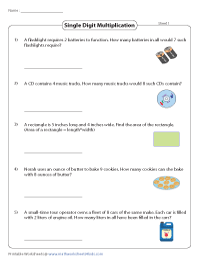Single-digit Multiplication Word Problems

The printable PDF worksheets presented here involve single-digit multiplication word problems. Each worksheet carries five word problems based on day-to-day scenarios.Multiplication Word Problems: Two-digit times Single-digit

The word problems featured here require a grade 3 learner to find the product by multiplying a two-digit number by a single-digit multiplier.Multiplication Word Problems: Two-digit times Two-digit

The worksheets presented here involve multiplication of two-digit numbers. Read the word problems and find the product. Apply long multiplication (also known as column multiplication) method for easy calculation.Theme Based Word Problems

Our engaging theme-based pdf worksheets help young minds understand the fundamentals of multiplication. Answer the word problems based on three fascinating themes - Winter Season, Ice rink and Library.Multiplication Word Problems: Three-digit times Two-digit

Read the word problems featured in these printable worksheets for grade 4 and find the product of three-digit and two-digit numbers. Write down your answers and use the answer key below to check if they are right.Three-digit Multiplication Word Problems

Solve these well-researched word problems that involve three-digit multiplication. Perform multiplication operation and carry over numbers carefully to find the product.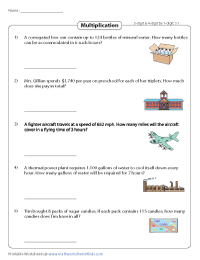Multiplication: Three or Four-digit times Single-digit

The word problems featured here are based on practical applications and fact-based situations. Multiply a three or four-digit number by a single-digit multiplier to find the correct product.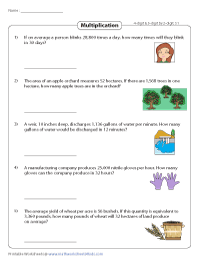Multi-digit Word Problems: Multiplying Large Numbers

Sharpen your skills by solving these engaging multi-digit word problems for grade 5. Apply long multiplication method to solve the problems. Use the answer key to check your answers.

Related Worksheets

» Subtraction Word Problems

» Division Word Problems

» Word Problems

» Multiplication

Become a Member

Membership Information

What's New?

Printing Help

TestimonialMembers have exclusive facilities to download an individual worksheet, or an entire level.## Multiplication Word ProblemsExcerpted fromThese workbooks have been compiled and tested by a team of math experts to increase your child's confidence, enjoyment, and success at school. Fourth Grade Math Made Easy provides practice at all the major topics for Grade 4 with emphasis on multiplication and division of larger numbers. It includes a review of Grade 3 topics and a preview of topics in Grade 5. It also includes Times Tables practice. Learn how the workbook correlates to the Common Core State Standards for mathematics.## Related Resources## Teaching Multiplication Word Problems (Grade 4)

Students often wonder why they’re learning a given math concept and word problems come in handy to help them realize the practical application of what is being taught. However, teaching word problems can be challenging, both for teachers and students.

This is why we’ll share simple tips on teaching multiplication word problems (grade 4) for teachers and homeschooling parents. Read on to learn more.

## What Are Multiplication Word Problems?

Multiplication word problems are math problems that represent actual situations from the real world and involve multiplication.

However, when solving multiplication word problems, children also come across multi-step word problems . These are problems with more than one operation, such as addition, subtraction, multiplication, or division.

## How to Solve Multiplication Word Problems (Grade 4)

Explain to students that the trickiest part of any word problem is understanding what is being asked. If they don’t know what they’re being asked to do, it will be very difficult to solve the math problem.

Sometimes, a word problem involves so many details that the key question gets lost. Explain to students that this is why it’s crucial to identify what’s important in a given word problem and what isn’t.

Encourage students to use a highlighter when solving multiplication word problems to highlight the keywords, key numbers, and key operations in a word problem. Provide an easy example where this can be applied, such as:

Jeremy has 7 caramels and he has 5 times as many marshmallows . How many more marshmallows than caramels does he have ?Explain that the thing we’re trying to find out is how many more marshmallows than caramels Jeremy has – this is the main question that students are asked. To answer the question, explain that they’ll use the underlined key information and perform two steps.

The first step is to figure out how many marshmallows Jeremy has; he has 5 x 7 as many marshmallows or 35 marshmallows . In other words, the first step is multiplication.

In the second step, once children are aware that Jeremy has 35 marshmallows and 7 caramels, they can easily find out how many more marshmallows than caramels he has by subtracting the total number of caramels from the total number of marshmallows, that is:

35 – 7 = 28

So Jeremy has 28 more marshmallows than caramels, which children could find out by engaging in two steps – multiplication and subtraction.

## Move on to ‘Wordier’ Multiplication Word Problems

Don’t jump into extremely wordy problems right away, but gradually transition to word problems of moderate difficulty that involve several steps, such as:

Mr. Conner bought 15 packets of pencils for his students. 5 of the packets had 6 pencils and the other 10 of the packet had 8 pencils . How many pencils did Mr.Conner buy in all ?Explain to students that after they underline the important information, they need to assess which steps they need to take. For starters, make sure they understand they’re trying to find out the answer to the key question – how many pencils Mr. Conner bought in total?

To be able to answer this question, explain to them that they’ll use the key information that’s highlighted in the word problem as we did before, taking one step at a time. Point out that we know there are 5 packets of pencils that have 6 pencils each, which means:

5 x 6 = 30 pencils

The other key information they have is that there are 10 packets of pencils with 8 pencils each, that is:

10 x 8 = 80 pencils

Finally, point out that we’ll add the total number of pencils in 5 of the packets and in 10 of the packets, or:

30 + 80 = 110 pencils

So, the answer to “how many pencils Mr. Conner bought in total” is 110 pencils. This multi-step problem involved two multiplication steps and one addition step to get the right answer.

## Activities to Practice Multiplication Word Problems (Grade 4)

Use this Assignment Worksheet (Members Only) to further reinforce children’s skills in solving multiplication word problems. The worksheet contains a dozen multiplication word problems that contain relatable and fun examples.

For instance, in part A of the worksheet, students are asked to solve a number of challenges connected to 4th graders in Mr. Vincent’s class that went to an amusement park last week, bought ice cream, and visited a haunted house.

To prepare for this activity, print out one copy of the worksheet (Members Only) per student. Make sure to bring a sufficient number of markers with different colors that students can use to highlight the key information in the math problems.

Explain to students that they’ll work in pairs of two to solve the word problems. Hand out the worksheets and markers to each pair. After a pair is done, they present their worksheet in front of the entire class by explaining each step they took to solve the given multi-step word problem.

## Online Math Activity

Use this online math activity from Khan Academy to solve multiplication word problems. There’s a big variety of multi-step word problems that involve multiplication as a step that children will definitely enjoy.

Make sure that there’s a suitable technical device for each student (such as a laptop, iPad, or even a phone) so that they play individually. Since these online word problems can be done individually, they’re also a great resource for parents who are homeschooling their children.

Each question even contains a hint or a video in case the child gets stuck.

## Before You Leave…

We hope you’ve enjoyed our tips on teaching math! If you’re looking for more math materials for kids of all ages, sign up for a membership on Math Teacher Coach or head over to our blog – you’ll find plenty of resources that will make teaching math easier than ever!

Unit 3 – Multi-Digit Whole Number Multiplication

• 3-1 Area and Perimeter Models
• 3-2 Multiplying by 10, 100, and 1,000
• 3-3 Multiplying Two-Digit by One-Digit Number
• 3-4 Multiplying Four-Digit by One-Digit Number
• 3-5 Multiplying Two-digit by Two-digit Number
• 3-6 Solve Multiplication Word Problems (Two-Step and Multi-Step)

• Click to share on Twitter (Opens in new window)
• Click to share on Facebook (Opens in new window)

Notify me of follow-up comments by email.

Notify me of new posts by email.## How to Solve Multiplication Word Problems## Word Problems: Multiplying Two Numbers

If there are 5 people in Karen's family, and if each person ate 4 pancakes , how many pancakes did Karen's family eat in all ?## Steps to Solving Word Problems

Pancakes each person had = 4
Number of people (Groups) = 5
Total pancakes (Product) = ?
5 times 4 = ? or 5 × 4 = ?
5 × 4 = 5 + 5 + 5 + 5 = 20
Karen's family ate 20 pancakes in all.
25 × 6 = ? 25 apartments × 6 people per = ? Total residents25 × 6 = 150
150 people can live in the apartment building in all.

## Word Problems: Multiplying Three Numbers

If, every week, Andrea sells 5 eggs each to 7 customers, how many eggs does she sell in 8 weeks?5 × 7 × 8 = ?
5 × 7 × 8 = 35 × 85 × 7 × 8 = 280
Andrea sold 280 eggs in 8 weeks.## Learn Spelling

Learn grammar, learn handwriting.Algebra & Pre-Algebra

Comparing Numbers

Daily Math Review

Division (Basic)

Division (Long Division)

Hundreds Charts

Measurement

Multiplication (Basic)

Multiplication (Multi-Digit)

Order of Operations

Place Value

Probability

Skip Counting

Subtraction

Telling Time

Word Problems (Daily)

More Math Worksheets

Cause & Effect

Fact & Opinion

Fix the Sentences

Graphic Organizers

Synonyms & Antonyms

Writing Prompts

Writing Story Pictures

Writing Worksheets

More ELA Worksheets

Consonant Sounds

Vowel Sounds

Consonant Blends

Consonant Digraphs

Word Families

More Phonics Worksheets

## Early Literacy

Build Sentences

Sight Word Units

Sight Words (Individual)

More Early Literacy

Punctuation

Subjects and Predicates

More Grammar Worksheets

## Spelling Lists

More Spelling Worksheets

## Chapter Books

Charlotte's Web

Magic Tree House #1

Boxcar Children

More Literacy Units

Animal (Vertebrate) Groups

Animal Articles

Butterfly Life Cycle

Electricity

Matter (Solid, Liquid, Gas)

Simple Machines

Space - Solar System

More Science Worksheets

## Social Studies

Maps (Geography)

Maps (Map Skills)

More Social Studies

Thanksgiving

Christmas Worksheets

New Year's Worksheets

Winter Worksheets

More Holiday Worksheets

## Puzzles & Brain Teasers

Brain Teasers

Mystery Graph Pictures

Number Detective

Lost in the USA

More Thinking Puzzles

## Teacher Helpers

Teaching Tools

Award Certificates

More Teacher Helpers

## Pre-K and Kindergarten

Alphabet (ABCs)

Numbers and Counting

Shapes (Basic)

More Kindergarten## Worksheet Generator

Word Search Generator

Multiple Choice Generator

Fill-in-the-Blanks Generator

More Generator Tools

Full Website Index

## Multiple-Step Word Problems

These multiple-step word problems require students to use reasoning and critical thinking skills to determine how each problem can be solved.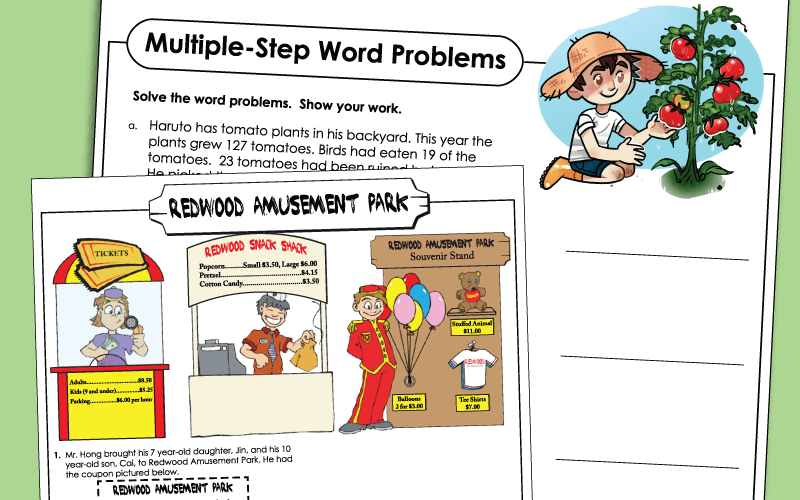## Basic (Grades 2 - 3)Logged in members can use the Super Teacher Worksheets filing cabinet to save their favorite worksheets.## Intermediate (Grades 3 - 4)

These word problems are sorted by type: addition, subtraction, multiplication, division, fractions and more.

These worksheets, sorted by grade level, cover a mix of skills from the curriculum.

S.T.W. has thousands of worksheets. Visit the full math index to find them all, sorted by topic.

## Sample Images of Our Printables## All Formats

Resource types, all resource types, multiplication word problems 4th grade.

• Rating Count
• Price (Ascending)
• Price (Descending)
• Most Recent## 4th Grade Multi-Step Word Problems Worksheets: Add, Subtract, Multiply , Divide..## 4th Grade Multiplication Word Problems Activity: Escape Room Math Breakout Game## 1- & 2-Step Multiplication & Division Word Problem Task Cards | 4th Grade | 4.4H## 4th Grade Multiplication and Division Word Problems {No Prep Intervention}## Multiplication Word Problems 4th Grade Multiplicative Comparison• Easel Activity## Word Problems - Bar Models Multiplication & Division 3rd 4th Grade Math Centers## 2-Digit by 2-Digit Multiplication Word Problem Task Cards | 4th Grade | TEK 4.4C## Multiplication Division 2 Steps Word Problems 3rd 4th Grade (Bar Models)## Multiply by 10, 100, and 1000 Word Problems 4th Grade## 4th Grade Word Problems Multiplication Division Math Classroom Transformation## 4th Grade Multiplication and Division Word Problems (Interactive Notebook)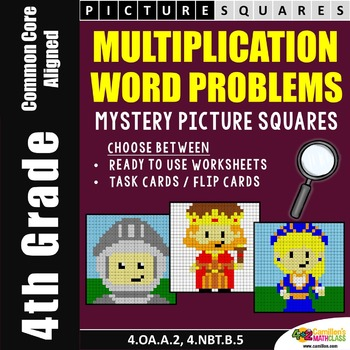## 4th Grade Multiplication Practice - Word Problems , Area, and Partial Products## 4th Grade Multi Step Word Problems Multiplication Division Task Cards DIGITAL## 4th Grade Multiplication and Division Word Problems Worksheets and DIGITAL## 4th Grade CGI Multiplication Word Problems and Discussion Guide Fall BUNDLE## Multiplication Word Problems for 2nd- 4th Grades : QR Code for Self-Check## Digital Math Pixel Art 4th Grade Math Review Multiplication , Word Problems## Thanksgiving Math Multiplication Word Problems for 4th / 5th Grade## 4th Grade Multiplication Word Problems Circus Classroom Transformation Game## 4th Grade Multiplication , Division & Word Problems Math Review Game Show• We're hiring
• Help & FAQ
• Student privacy
• Tell us what you think• Kindergarten
• Learning numbers
• Comparing numbers
• Place Value
• Roman numerals
• Subtraction
• Multiplication
• Order of operations
• Drills & practice
• Measurement
• Factoring & prime factors
• Proportions
• Shape & geometry
• Data & graphing
• Word problems
• Children's stories
• Leveled Stories
• Context clues
• Cause & effect
• Compare & contrast
• Fact vs. fiction
• Fact vs. opinion
• Main idea & details
• Story elements
• Conclusions & inferences
• Sounds & phonics
• Words & vocabulary
• Early writing
• Numbers & counting
• Simple math
• Social skills
• Other activities
• Dolch sight words
• Fry sight words
• Multiple meaning words
• Prefixes & suffixes
• Vocabulary cards
• Other parts of speech
• Punctuation
• Capitalization
• Cursive alphabet
• Cursive letters
• Cursive letter joins
• Cursive words
• Cursive sentences
• Cursive passages
• Grammar & Writing

• Word Problems
• Multiplication & division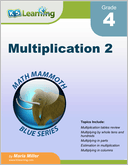## Multiplication & division word problems

Mixed multiplication & division.

These grade 4 math worksheets have mixed multiplication and division word problems . All numbers are whole numbers with 1 to 4 digits. Division questions may have remainders which need to be interpreted (e.g. "how many left over"). In the last question of each worksheet, students are asked to write an equation with a variable for the unknown quantity.These worksheets are available to members only.

## More word problem worksheets

Explore all of our math word problem worksheets , from kindergarten through grade 5.

What is K5?

K5 Learning offers free worksheets , flashcards  and inexpensive  workbooks  for kids in kindergarten to grade 5. Become a member  to access additional content and skip ads.Our members helped us give away millions of worksheets last year.

We provide free educational materials to parents and teachers in over 100 countries. If you can, please consider purchasing a membership (\$24/year) to support our efforts.

Members skip ads and access exclusive features.

This content is available to members only.

## Free Printable Multiplication Word Problems Worksheets for 4th Class

Multiplication Word Problems: Discover a collection of free printable worksheets tailored for Class 4 math teachers, focusing on enhancing students' problem-solving skills in multiplication scenarios.## Recommended Topics for you

• Multi-Digit Multiplication Word Problems
• One-Digit Multiplication Word Problems• kindergarten

## Explore Multiplication Word Problems Worksheets for class 4 by Topic

Explore other subject worksheets for class 4.

• Social studies
• Social emotional
• Foreign language

## Explore printable Multiplication Word Problems worksheets for 4th Class

Multiplication Word Problems worksheets for Class 4 are an essential tool for teachers to help their students develop a strong foundation in math. These worksheets provide a variety of math word problems that challenge students to apply their multiplication skills in real-life situations. By incorporating these worksheets into their lesson plans, teachers can effectively engage their students in understanding the importance of multiplication and its applications in everyday life. Furthermore, these Class 4 worksheets are designed to cater to different learning styles, ensuring that all students have the opportunity to grasp the concepts and excel in their math skills. With a wide range of problems and scenarios, Multiplication Word Problems worksheets for Class 4 are a valuable resource for teachers looking to enhance their students' mathematical abilities.

Quizizz is an innovative platform that offers a plethora of resources for teachers, including Multiplication Word Problems worksheets for Class 4, as well as other math-related materials. This platform allows teachers to create engaging quizzes and interactive lessons, making learning math more enjoyable and effective for students. In addition to the Class 4 worksheets, Quizizz also offers resources for other grade levels and subjects, ensuring that teachers have access to a comprehensive library of educational materials. The platform's user-friendly interface and customizable features make it an invaluable tool for educators looking to improve their students' performance in math and other subjects. By incorporating Quizizz into their teaching strategies, teachers can provide their students with a well-rounded and engaging learning experience that fosters academic success.#### IMAGES

1. Multiplication Word Problems 4Th Grade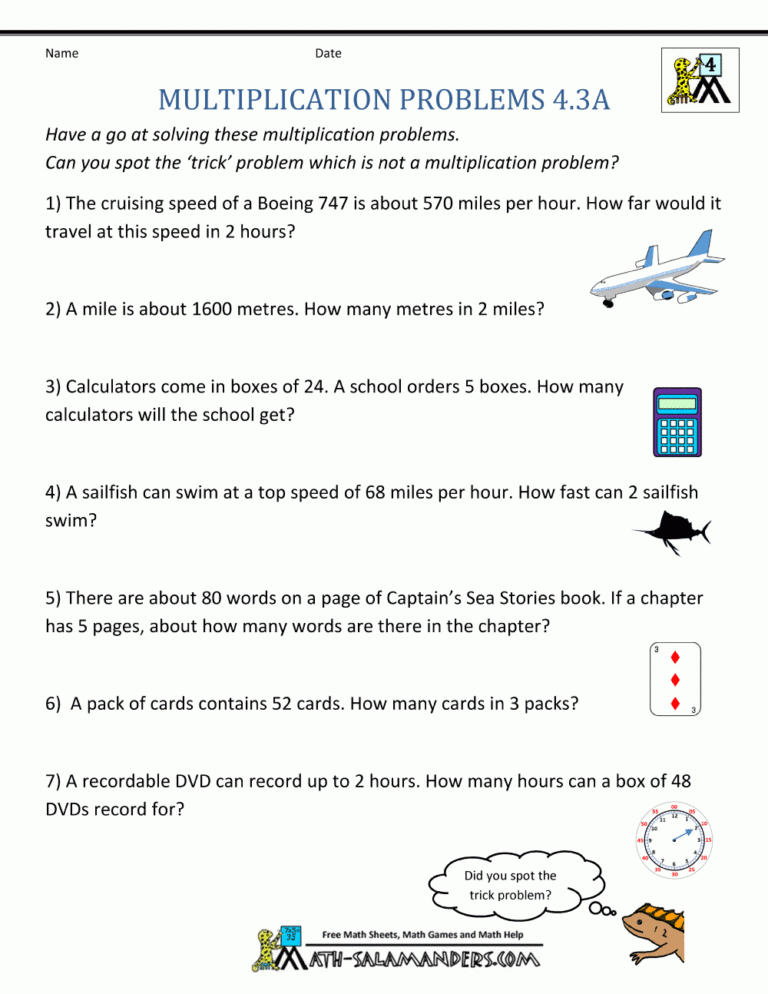2. multiplication word problems 4th grade worksheets 3 free math 43. Multiplication Word Problems 4th Grade4. 4th grade word problem worksheets printable k5 learning5. Pin on Back-to-School Rules6. 4th Grade Two Digit Multiplication Worksheets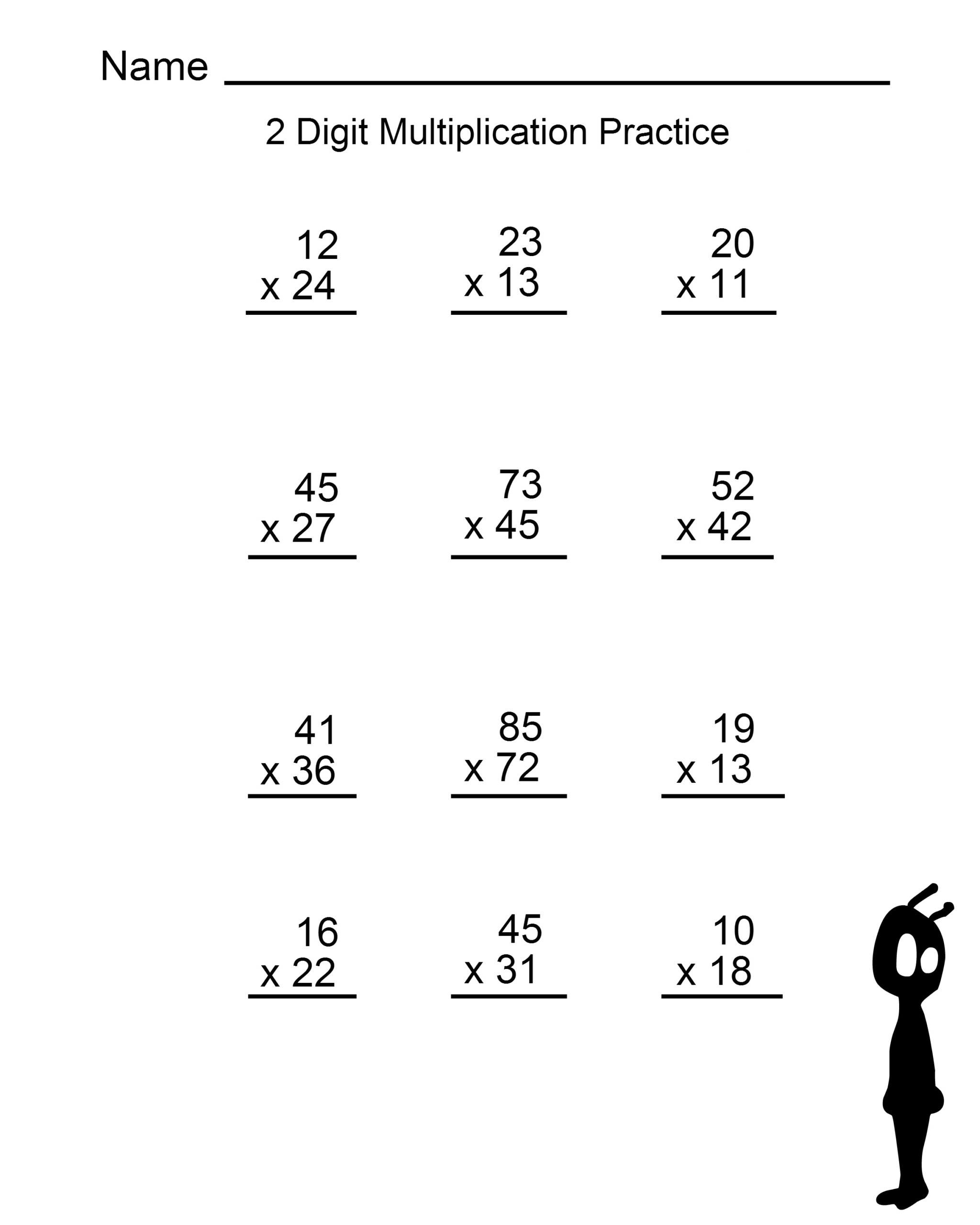#### VIDEO

1. Multiplication Word Problems with Part Part Whole

2. Multiplication Word Problem 11/1/2023

3. Solve Word Problems 3rd Grade

4. Part two: multiplication word problems for primary 3

5. Word Problems of Multiplication Class IV part 5 by Meenu O P

6. Multiplication word problem, concept of addition!!!

1. Multiplication Word Problems 4th Grade

Welcome to our Multiplication Word Problems for 4th Grade. Here you will find our range of printable multiplication problems which will help your child apply and practice their multiplication and times tables skills to solve a range of 'real life' problems at a 4th grade level. Quicklinks to ... 4th Grade Multiplication Problems Worksheets

2. Multiplication Word Problems Worksheets

Read the word problems featured in these printable worksheets for grade 4 and find the product of three-digit and two-digit numbers. Write down your answers and use the answer key below to check if they are right. Three-digit Multiplication Word Problems Solve these well-researched word problems that involve three-digit multiplication.

3. 4th grade word problem worksheets

4th grade word problem worksheets including multiplication and division word problems, fraction and decimal word problems, measurement word problems and mixed word problems. No login required.

4. IXL

IXL plans. Virginia state standards. Textbooks. Test prep. Awards. Improve your math knowledge with free questions in "Multiplication word problems" and thousands of other math skills.

5. Browse Printable 4th Grade Multiplication Word Problem Worksheets

Multiplication Word Problems Show interactive only Sort by Math Mixed Review Part 1: Flying Through Fourth Grade Worksheet Wild Word Problems: Multiplication Interactive Worksheet Word Problem Assessment: Flying Through Fourth Grade Worksheet Multiplication: Word Problems (Part One) Worksheet Winter Multiplication and Division Word Problems

Multiplication worksheets Multiplying numbers in columns requires lots of practice. Our grade 4 worksheets range in difficulty from 2 x 1 digits to 3 x 3 digits . These worksheets complement our grade 4 mental multiplication worksheets which focus on practicing "in your head" multiplication skills. Sample grade 4 multiplication in columns worksheet

7. 50+ Multiplication Word Problems worksheets for 4th Grade on Quizizz

Free Printable Multiplication Word Problems Worksheets for 4th Grade Multiplication Word Problems: Discover a collection of free printable worksheets tailored for Grade 4 math teachers, focusing on enhancing students' problem-solving skills in multiplication scenarios. grade 4 Multiplication Word Problems Recommended Topics for you

8. Search Printable 4th Grade Multiplication Worksheets

Math Mixed Review Part 1: Flying Through Fourth Grade. Worksheet. Multiplication Practice Part 2: Write the Missing Number. Interactive Worksheet. Multiplication and Division Practice. Interactive Worksheet. Mittens and Math: Two-Digit Multiplication Practice. Interactive Worksheet. Multiplication: Star Arrays.

9. Multiplication Word Problems Worksheet (Grade 4)

The word problems in this worksheet provide an opportunity for children to apply their multiplication skills to real-world problems. Students will practice multiplying by three-digit and four-digit numbers. ... Fourth Grade Math Made Easy provides practice at all the major topics for Grade 4 with emphasis on multiplication and division of ...

Math Multiplication & Division Fourth Grade - Word Problems Word Problems Two-step Word Problem Multiplication Challenge Cards for 3rd-5th Grade 4.8 (6 reviews) Multiplication Word Problems Code Hunter Activity 5.0 (10 reviews) Word Problems Using Multiplication and Division Activity 4.6 (7 reviews)

Learn fourth grade math—arithmetic, measurement, geometry, fractions, and more. This course is aligned with Common Core standards. ... Compare with multiplication word problems; Multiply by 1-digit numbers: Quiz 1; Multiply 1-digit numbers by 10, 100, and 1000;

12. Compare with multiplication word problems

4th grade. Course: 4th grade > Unit 3. Lesson 1: Comparing with multiplication. Multiply by 1-digit numbers: FAQ. Comparing with multiplication. Comparing with multiplication and addition: giraffe. ... Compare with multiplication word problems. Google Classroom. Problem.

13. Teaching Multiplication Word Problems (Grade 4)

How to Solve Multiplication Word Problems (Grade 4) Start With Simple Multiplication Word Problems Explain to students that the trickiest part of any word problem is understanding what is being asked. If they don't know what they're being asked to do, it will be very difficult to solve the math problem.

14. Multiplication and division word problems

The following collection of free 4th grade maths word problems worksheets cover topics including addition, subtraction, multiplication, division, mixed operations, fractions, and decimals.

16. Multiplication Word Problems

To solve any word problem, follow these steps: STEP 1: Read the problem carefully. Note what numbers are given, and what number needs to be found. STEP 2: Write an equation using the numbers given. STEP 3: Use this equation to find the answer to the problem. STEP 4: Write your final answer as a complete sentence, so people can understand what ...

17. Word Problems Grade 4 Multiplication

11 grade 4 multiplication questions to develop reasoning and problem solving skills. 10 multiplication questions with an additional challenge question. Answers included with reference to the associated standard. Questions include factor pairs, multiplication grids and multiples.

18. Multi-step word problems with whole numbers

Multi-step word problems with whole numbers. Google Classroom. After collecting eggs from his chickens, Dale puts the eggs into cartons to sell. Dale fills 15 cartons and has 7 eggs left over. Each carton holds 12 eggs.

19. Multiple-Step Word Problem Worksheets

Multiple Step, Basic #1 FREE Solve each of the multi-step word problems on this page. Problems contain basic numbers of 20 or less. Each problem can be solved without knowledge of multiplication or division. 2nd and 3rd Grades View PDF Multiple Step, Basic #2 These multi-step questions can be calculated using only addition and subtraction.

20. multiplication word problems 4th grade

This resource includes 20 multiplication and division word problems for use in interactive notebooks. The word problems are aligned with 4th grade math standards and include multiplying word problems (10 problems) and dividing word problems (10 problems). Multiplication: 3-digit by 1-digit, 4-digit by 1-digit, and 2-digit by 2-digitDivision: 3 ...

21. Mixed multiplication and division word problems for grade 4

These grade 4 math worksheets have mixed multiplication and division word problems. All numbers are whole numbers with 1 to 4 digits. Division questions may have remainders which need to be interpreted (e.g. "how many left over").

22. 50+ Multiplication Word Problems worksheets for 4th Class on Quizizz

Multiplication Word Problems: Discover a collection of free printable worksheets tailored for Class 4 math teachers, focusing on enhancing students' problem-solving skills in multiplication scenarios. class 4 Multiplication Word Problems Recommended Topics for you Multi-Digit Multiplication Word Problems One-Digit Multiplication Word Problems

23. IXL

Multiplication and division word problems 5ND Share skill Learn with an example or Watch a video Questions answered 0 Time elapsed SmartScore out of 100 IXL's SmartScore is a dynamic measure of progress towards mastery, rather than a percentage grade. It tracks your skill level as you tackle progressively more difficult questions.

24. Browse Printable 4th Grade Multi-Digit Multiplication Word Problem

Math Mixed Review Part 1: Flying Through Fourth Grade. Worksheet. Wild Word Problems: Multiplication. Interactive Worksheet. Winter Multiplication and Division Word Problems. Interactive Worksheet. Merchandise Multiplication. Worksheet. Read, Draw, Write!